Tapping into mathematics

Start this free course now. Just create an account and sign in. Enrol and complete the course for a free statement of participation or digital badge if available.

Free course

# 2.4 Bracket keys

A way of forcing a calculator to perform a calculation in a different order to that given in Section 2.3 is to use the bracket keys. For example the following sequence, on a scientific or graphics calculator:

7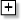2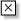4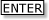normally produces the answer 15. The reason is that the calculator performs the multiplication (2 × 4) before the addition (7 + 8). Now, suppose you wish to perform the addition before the multiplication. The way to override the calculator's pre-programmed choice to do multiplication before addition is to place the addition part of the sequence in brackets. Press: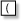72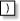4This gives the answer 36 (that is, 9 × 4).

## Exercise 3: Guess and press

Look at the calculator sequences below and make an intelligent guess as to the answer that your calculator would give. Then press the sequence on your calculator and see what the actual result is. Note down any comments on your results. (If your calculator has an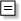key then use it instead of).

• (a) 24• (b) 2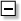3• (c) 2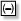3• (d) 345• (e) 46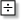2• (f) 34219• (g)6• (h) 40• (i) 3(24)(42)(a) 6; (b) 1; (c) 1; (d) 23; (e) 7; (f) 10; (g) 60; (h) error – you can't divide by 0; (i) 9.

Note multiplication and division are done first, unless there are brackets, in which case the calculation inside the brackets is done first.

MU120_4MCAL